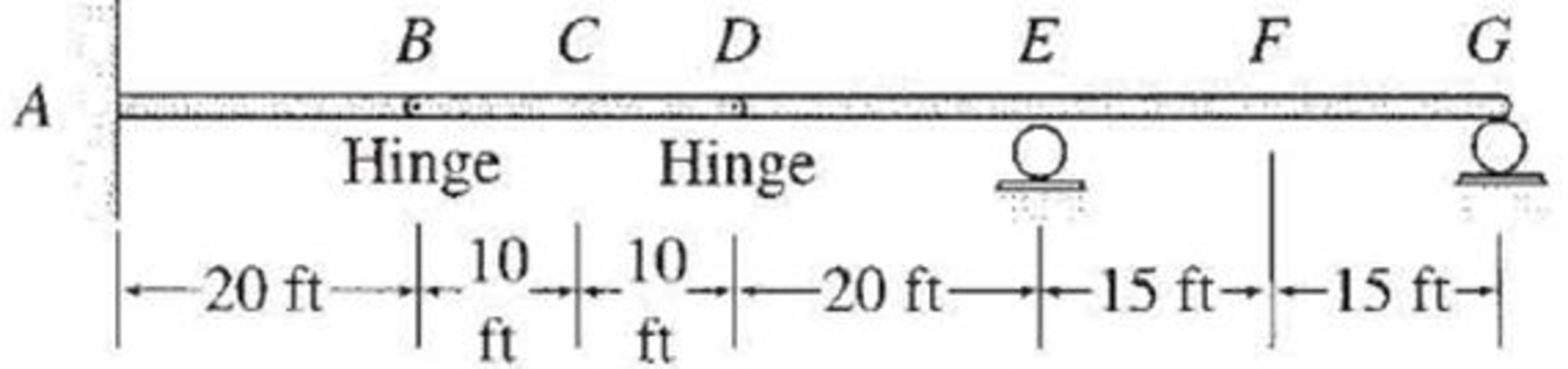# Draw the influence lines for the reaction moment at support A and the vertical reactions at supports A , E , and G of the beam shown in Fig. P8.29. FIG. P8.29, P8.30

#### Solutions

Chapter
Section
Chapter 8, Problem 29P
Textbook Problem
32 views

## Draw the influence lines for the reaction moment at support A and the vertical reactions at supports A, E, and G of the beam shown in Fig. P8.29.FIG. P8.29, P8.30

To determine

Draw the influence lines for the reaction moment at support A.

Draw the influence lines for the vertical reactions at supports A, E, and G.

### Explanation of Solution

Calculation:

Use Muller-Breslau's principle.

Remove the restraint of fixed support at A by replace the fixed support at A to a hinged support.

Next apply a small rotation θ in the negative (counterclockwise) direction at A to obtain the deflected shape of the beam.

Sketch the deflected shape of the released structure when the fixed support replaced by hinged support is released as shown in Figure 1.

The shape of the influence line is the same as the deflected shape of the released structure.

Sketch the general shape of the influence line for moment (MA) at support A as shown in Figure 2.

The influence line ordinate at A is zero. Hence, find the influence line ordinate for MA at B by apply a 1 k at B.

Sketch the free body diagram of beam when 1 kN load at B as shown in Figure 3.

Refer Figure 3.

Find the reaction (Ay) at A.

Consider force equilibrium of member AB.

Take upward force as positive and downward as negative.

ΣFy=0Ay1=0Ay=1k

Find the moment at B.

ΣMB=0MB=(Ay×20)MB=(1×20)MB=20k-ft

Thus, the value of influence line ordinate at B is 20k-ft/k.

The value of influence line ordinate in the different points on the beam determined by geometry (similar triangle) of the influence line

Similarly calculate the influence line ordinate for MA in different points on beam and summarize the values as in Table 1.

 x (ft) Points Influence line ordinate of MA(k-ft/k) 0 A 0 20 B 20 30 C 10 40 D 0 60 E 0 75 F 0 90 G 0

Sketch the influence line diagram for the reaction moment at support A using Table 1 as shown in Figure 4.

Replace the fixed support at A by a roller that prevents the rotation and displacement along horizontal but not in displacement along vertical.

Sketch the deflected shape of the released structure when the fixed support replaced by roller as shown in Figure 5.

The shape of the influence line is the same as the deflected shape of the released structure.

Sketch the general shape of the influence line for vertical reaction (Ay) at supports A as shown in Figure 6.

Apply 1 k unit load at point A.

Find the influence line ordinate (AA) for Ay at A.

Generally the influence line ordinate (AA’) at A is 1 k/k when 1 k load is placed at A. The values of influence line ordinate in the different points on the beam are determined by geometry (similar triangle) of the influence line.

Find the influence line ordinate (BB) for Ay at B.

The value of influence line ordinate for Ay at A and B are equal.

Hence, the influence line ordinate (BB) for Ay at B is 1 k/k.

Similarly calculate the influence line ordinate for Ay in different points on beam and summarize the values as in Table 2.

 x (ft) Points Influence line ordinate of Ay(k/k) 0 A 1 20 B 1 30 C 12 40 D 0 60 E 0 75 F 0 90 G 0

Sketch the influence line diagram for the vertical reactions at supports A using Table 2 as shown in Figure 7.

Remove the roller support at E from the given beam and apply a small displacement Δ. Due to the displacement, the structure released in which the deflected shape of the released structure of the beam is drawn

### Still sussing out bartleby?

Check out a sample textbook solution.

See a sample solution

#### The Solution to Your Study Problems

Bartleby provides explanations to thousands of textbook problems written by our experts, many with advanced degrees!

Get Started

Find more solutions based on key concepts
In your own words, explain the water cycle.

Engineering Fundamentals: An Introduction to Engineering (MindTap Course List)

Define dirty data, and identify some of its sources.

Database Systems: Design, Implementation, & Management

What is a managerial control?

Management Of Information Security

What is Port Address Translation (PAT) and how does it work?

Principles of Information Security (MindTap Course List)

What is clickjacking?

Enhanced Discovering Computers 2017 (Shelly Cashman Series) (MindTap Course List)

If your motherboard supports ECC DDR3 memory, can you substitute non-ECC DDR3 memory?

A+ Guide to Hardware (Standalone Book) (MindTap Course List)